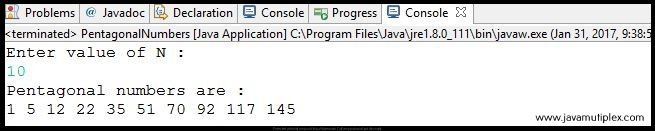## Problem Description :

Write a program in Java that generates first N pentagonal numbers.First N Pentagonal numbers

## Concept :

We can generate Nth pentagonal number using below formula.
N(3N-1)/2
For example -  first 6 pentagonal numbers are 1, 5, 12, 22, 35, 51.Pentagonal Numbers

## Output :Output - How to generate first n pentagonal numbers in Java?

## References :

https://en.wikipedia.org/wiki/Pentagonal_number

Thank you friends, I hope you have clearly understood the solution of this problem. If you have any doubt, suggestion or query please feel free to comment below. You can also discuss this solution in our forum.

Tags : Pentagonal numbers in Java, Solution in Java, Mathematics problems, for loop, if else statement.How to generate first N Pentagonal numbers in Java?Reviewed by Rohit Agarwal on 1/31/2017 Rating: 5